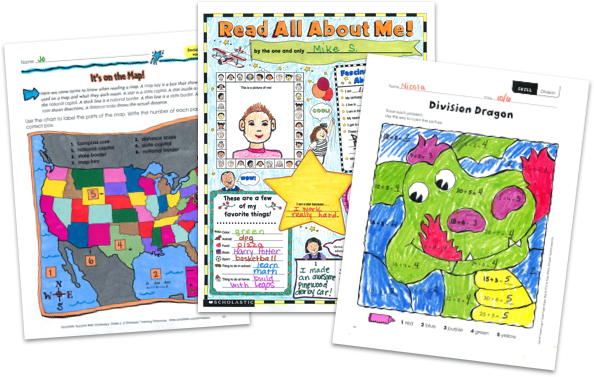MY FILE CABINET# Fraction Worksheets and Lesson Plans From Scholastic TeachablesScholastic Teachables (formerly Scholastic Printables) has more than 750 fractions worksheets and fractions lesson plans for grades 1 to 8! Sign up today and get instant online access to printable fractions worksheets, lesson plans, practice pages, and more to cover all levels of skills in working with fractions. Plus, you'll get access to more than 30,000 award-winning printables and leveled learning collections in math and reading to reach every learner.

Scholastic Teachables (formerly Scholastic Printables) has more than 750 fractions worksheets and fractions lesson plans for grades 1 to 8! Sign up today and get instant online access to printable fractions worksheets, lesson plans, practice pages, and more to cover all levels of skills in working with fractions. Plus, you'll get access to more than 30,000 award-winning printables and leveled learning collections in math and reading to reach every learner.

## Scholastic's Fraction Worksheets and Lesson Plans Cover:

• Common Fractions
• Comparing Fractions
• Converting Fractions to Decimals
• Converting Fractions to Percents
• Denominators
• Dividing Fractions
• Equal Fractions
• Equal Parts
• Fraction Basics
• Fraction Operations
• Fractional Parts
• Mixed Numbers and Improper Fractions
• Multiplying Fractions
• Numerators
• Ordering Fractions
• Parts of a Whole
• Real-World Math
• Reducing Fractions
• Simple Fractions
• Simplifying Fractions
• Subtracting Fractions
• Word Problems

## Sample Fraction Worksheets and Lesson Plans:

More than 750 printable fraction worksheets and lesson plans cover all grade levels and skills! Here's a sample of worksheets and lesson plans for you to try in your class—FREE with a 30-day trial or subscription.

## Scholastic's Fraction Worksheets and Lesson Plans Cover:

• Common Fractions
• Comparing Fractions
• Converting Fractions to Decimals
• Converting Fractions to Percents
• Denominators
• Dividing Fractions
• Equal Fractions
• Equal Parts
• Fraction Basics
• Fraction Operations
• Fractional Parts
• Mixed Numbers and Improper Fractions
• Multiplying Fractions
• Numerators
• Ordering Fractions
• Parts of a Whole
• Real-World Math
• Reducing Fractions
• Simple Fractions
• Simplifying Fractions
• Subtracting Fractions
• Word Problems
• Common Fractions
• Comparing Fractions
• Converting Fractions to Decimals
• Converting Fractions to Percents
• Denominators
• Dividing Fractions
• Equal Fractions
• Equal Parts
• Fraction Basics
• Fraction Operations
• Fractional Parts
• Mixed Numbers and Improper Fractions
• Multiplying Fractions
• Numerators
• Ordering Fractions
• Parts of a Whole
• Real-World Math
• Reducing Fractions
• Simple Fractions
• Simplifying Fractions
• Subtracting Fractions
• Word Problems

## Sample Fraction Worksheets and Lesson Plans:

More than 750 printable fraction worksheets and lesson plans cover all grade levels and skills! Here's a sample of worksheets and lesson plans for you to try in your class—FREE with a 30-day trial or subscription.## Additional Alphabet Worksheets (Subscription Required)

Try FREE for 30 days!

If you are not 100% satisfied cancel during the FREE trial and owe nothing. If you are not 100% satisfied cancel during the FREE trial and owe nothing.